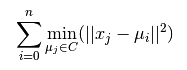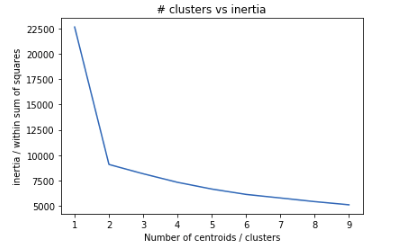Machine Learning

# K-Means model for Predicting Car quality[Case Study]

## Problem Statement :

To build a simple K-Means model for clustering the car data into different groups.

## Data details

==========================================
1. Title: Car Evaluation Database==========================================
The dataset is available at  “http://archive.ics.uci.edu/ml/datasets/Car+Evaluation
2. Sources:
(a) Creator: Marko Bohanec
(b) Donors: Marko Bohanec   (marko.bohanec@ijs.si)
Blaz Zupan      (blaz.zupan@ijs.si)
(c) Date: June, 19973. Past Usage:

The hierarchical decision model, from which this dataset is derived, was first presented in M. Bohanec and V. Rajkovic: Knowledge acquisition and explanation for multi-attribute decision making. In 8th Intl Workshop on Expert Systems and their Applications, Avignon, France. pages 59-78, 1988.

Within machine-learning, this dataset was used for the evaluation of HINT (Hierarchy INduction Tool), which was proved to be able to completely reconstruct the original hierarchical model. This, together with a comparison with C4.5, is presented in B. Zupan, M. Bohanec, I. Bratko, J. Demsar: Machine learning by function decomposition. ICML-97, Nashville, TN. 1997 (to appear)

4. Relevant Information Paragraph:

Car Evaluation Database was derived from a simple hierarchical decision model originally developed for the demonstration of DEX(M. Bohanec, V. Rajkovic: Expert system for decision making. Sistemica 1(1), pp. 145-157, 1990.). The model evaluates cars according to the following concept structure:

CAR                      car acceptability
. PRICE                  overall price
. . maint                price of the maintenance
. TECH                   technical characteristics
. . COMFORT              comfort
. . . doors              number of doors
. . . persons            capacity in terms of persons to carry
. . . lug_boot           the size of luggage boot
. . safety               estimated safety of the car

Input attributes are printed in lowercase. Besides the target concept (CAR), the model includes three intermediate concepts: PRICE, TECH, COMFORT. Every concept is in the original model related to its lower level descendants by a set of examples (for these examples sets see http://www-ai.ijs.si/BlazZupan/car.html).

The Car Evaluation Database contains examples with the structural information removed, i.e., directly relates CAR to the six input attributes: buying, maint, doors, persons, lug_boot, safety.
Because of known underlying concept structure, this database may be particularly useful for testing constructive induction and structure discovery methods.

5. Number of Instances: 1728
(instances completely cover the attribute space)

6. Number of Attributes: 6

7. Attribute Values:

maint        v-high, high, med, low
doors        2, 3, 4, 5-more
persons      2, 4, more
lug_boot     small, med, big
safety       low, med, high

8. Missing Attribute Values: none

9. Class Distribution (number of instances per class)

class      N          N[%]
—————————–
unacc     1210     (70.023 %)
acc        384     (22.222 %)
good        69     ( 3.993 %)
v-good      65     ( 3.762 %)

Tools to be used :

Numpy,pandas,scikit-learn

Python Implementation with code :

Import necessary libraries

Import the necessary modules from specific libraries.

```import os
import numpy as np
import pandas as pd
import numpy as np, pandas as pd
import matplotlib.pyplot as plt
from sklearn.cluster import KMeans
```

Use pandas module to read the bike data from the file system. Check few records of the dataset.

```data = pd.read_csv('data/car_quality/car.data',names=['buying','maint','doors','persons','lug_boot','safety','class'])
```
```buying    maint       doors      persons  lug_boot safety      class

0              vhigh      vhigh      2              2              small       low          unacc

1              vhigh      vhigh      2              2              small       med         unacc

2              vhigh      vhigh      2              2              small       high        unacc

3              vhigh      vhigh      2              2              med         low          unacc

4              vhigh      vhigh      2              2              med         med         unacc
```

Check few information about the data set

```data.info()
```
```<class 'pandas.core.frame.DataFrame'>
RangeIndex: 1728 entries, 0 to 1727
Data columns (total 7 columns):
maint       1728 non-null object
doors       1728 non-null object
persons     1728 non-null object
lug_boot    1728 non-null object
safety      1728 non-null object
class       1728 non-null object
dtypes: object(7)
memory usage: 94.6+ KB
```

The train data set has 1728 rows and 7 columns.
There are no missing values in the dataset

Identify the target variable

```data['class'],class_names = pd.factorize(data['class'])
```

The target variable is marked as class in the dataframe. The values are present in  string format. However the algorithm requires the variables to be coded into its equivalent integer codes. We can convert the string categorical values into a integer code using factorize method of the pandas library.

Let’s check the encoded values now.

```print(class_names)
print(data['class'].unique())
```
```Index([u'unacc', u'acc', u'vgood', u'good'], dtype='object')
[0 1 2 3]
```

As we can see the values has been encoded into 4 different numeric labels.
Identify the predictor variables and encode any string variables to equivalent integer codes

```data['buying'],_ = pd.factorize(data['buying'])
data['maint'],_ = pd.factorize(data['maint'])
data['doors'],_ = pd.factorize(data['doors'])
data['persons'],_ = pd.factorize(data['persons'])
data['lug_boot'],_ = pd.factorize(data['lug_boot'])
data['safety'],_ = pd.factorize(data['safety'])
```
```buying            maint       doors      persons  lug_boot safety      class

0              0              0              0              0              0              0              0

1              0              0              0              0              0              1              0

2              0              0              0              0              0              2              0

3              0              0              0              0              1              0              0

4              0              0              0              0              1              1              0

```

Check the data types now :

```data.info()
```
```<class 'pandas.core.frame.DataFrame'>
RangeIndex: 1728 entries, 0 to 1727
Data columns (total 7 columns):
maint       1728 non-null int64
doors       1728 non-null int64
persons     1728 non-null int64
lug_boot    1728 non-null int64
safety      1728 non-null int64
class       1728 non-null int64
dtypes: int64(7)
memory usage: 94.6 KB
```

Everything is now converted in integer form.
Select the predictor feature and select the target variable
In clustering there is no target variable as such. However clustering helps us to find a cluster which can be used as weak labels. These weak labels can bootstrap our supervised learning.
This technique is widely used for semi-supervised learning.
We need not worry about it as of now. You can just consider that y value will be used to validate the accuracy of weak labeling going ahead.

```X = data
y = data.iloc[:,-1]
```

Training / model fitting

```model = KMeans(n_clusters=4,random_state=123)
model.fit(X)
```

Check few parameters of the learnt cluster (Model parameters study):
Check the cluster centroids or means :
It should be 4 vectors or a matrix with 4 rows since the number of clusters we have fitted is 4.
Let’s check

```model.cluster_centers_
```
```[[1.76355748e+00 1.82429501e+00 8.37310195e-01 1.09327549e+00
1.05206074e+00 1.12581345e+00 8.45986985e-01 7.05422993e+00]
[1.72631579e+00 1.75438596e+00 2.50175439e+00 9.29824561e-01
9.64912281e-01 9.07017544e-01 3.19298246e-01 1.87719298e+00]
[5.00000000e-01 1.50000000e+00 1.50000000e+00 1.00000000e+00
1.00000000e+00 1.00000000e+00 1.94444444e-01 4.50000000e+00]
[2.18490566e+00 3.88679245e-01 4.98113208e-01 9.88679245e-01
9.84905660e-01 9.81132075e-01 2.45283019e-01 1.06581410e-14]]
```

Check the goodness of the cluster i.e. within sum of square of the model:

The KMeans algorithm clusters data by trying to separate samples in n groups of equal variance, minimizing a criterion known as the inertia or within-cluster sum-of-squares Inertia, or the within-cluster sum of squares criterion, can be recognized as a measure of how internally coherent clusters are.
The k-means algorithm divides a set of N samples X into K disjoint clusters C, each described by the mean j of the samples in the cluster. The means are commonly called the cluster “centroids”.
The K-means algorithm aims to choose centroids that minimise the inertia, or within-cluster sum of squared criterion:Inertia is not a normalized metric: we just know that lower values are better and zero is optimal. But in very high-dimensional spaces, Euclidean distances tend to become inflated (this is an instance of the so-called “curse of dimensionality”). Running a dimensionality reduction algorithm such as PCA prior to k-means clustering can alleviate this problem and speed up the computations.

```model.inertia_
```
```9447.907008065738
```

Lesser this number better is the model fit.
Check the quality of the weak classification by the model

```labels = model.labels_
# check how many of the samples were correctly labeled
correct_labels = sum(y == labels)
print("Result: %d out of %d samples were correctly labeled." % (correct_labels, y.size))

```
```Result: 456 out of 1728 samples were correctly labeled.
correct 0.26 classification
```

We have achieved a weak classification accuracy of 26% by our unsupervised model. Not Bad.

!!!
How do we know value of optimal value of K

The value of k basically means that in how many clusters you can separate out the most homogeneous data. Choosing a large value of K will lead to greater amount of execution time. Selecting the small value of K might or might not give a good fit. There is no such guaranteed way to find the best value of K. However we can run few experiments and find the value of each model’s inertia and plot it on a graph. This plot is also known as elbow plot. We basically try to find the value of k from where there is major shift in value of inertia.

Let’s plot the graph.

```from mpl_toolkits.mplot3d import Axes3D

def elbow_plot(data, maxK=40, seed_centroids=None):
"""
parameters:
- data: pandas DataFrame (data to be fitted)
- maxK (default = 10): integer (maximum number of clusters with which to run k-means)
- seed_centroids (default = None ): float (initial value of centroids for k-means)
"""
sse = {}
for k in range(1, maxK):
print("k: ", k)
if seed_centroids is not None:
kmeans = KMeans(n_clusters=k, max_iter=500, n_init=100, random_state=0, init=np.reshape(seeds, (k,1))).fit(data)
data["clusters"] = kmeans.labels_
else:
kmeans = KMeans(n_clusters=k, max_iter=300, n_init=100, random_state=0).fit(data)
data["clusters"] = kmeans.labels_
# Inertia: Sum of distances of samples to their closest cluster center
sse[k] = kmeans.inertia_
plt.figure()
plt.plot(list(sse.keys()), list(sse.values()))
plt.show()
return

elbow_plot(X, maxK=10)

```By the plot we can see that there is a kink at k=2. Hence k =2 can be considered as good number of cluster to cluster this data.
Let’s check the inertia and weak classification with number of cluster = 2.

```model = KMeans(n_clusters=2,random_state=123)
model.fit(X)
labels = model.labels_
# check how many of the samples were correctly labeled
correct_labels = sum(y == labels)

print("Result: %d out of %d samples were correctly labeled." % (correct_labels, y.size))
print("correct %.02f classification " % (correct_labels/float(y.size)))

```
```Result: 869 out of 1728 samples were correctly labeled.
correct 0.50 classification
```

Woah !!! our weak labeling is as good as a weak supervised classification model.

### Time Complexity

K-means is linear in the number of data objects i.e. O(n), where n is the number of data objects. The time complexity of most of the hierarchical clustering algorithms is quadratic i.e. O(n2). Therefore, for the same amount of data, hierarchical clustering will take quadratic amount of time. Imagine clustering 1 million records?

### Shape of Clusters

K-means works well when the shape of clusters are hyper-spherical (or circular in 2 dimensions). If the natural clusters occurring in the dataset are non-spherical then probably K-means is not a good choice.

### Repeatability

K-means starts with a random choice of cluster centers, therefore it may yield different clustering results on different runs of the algorithm. Thus, the results may not be repeatable and lack consistency. However, with hierarchical clustering, you will most definitely get the same clustering results.

Off course, K-means clustering requires prior knowledge of K (or number of clusters), whereas in hierarchical clustering you can stop at whatever level (or clusters) you wish.